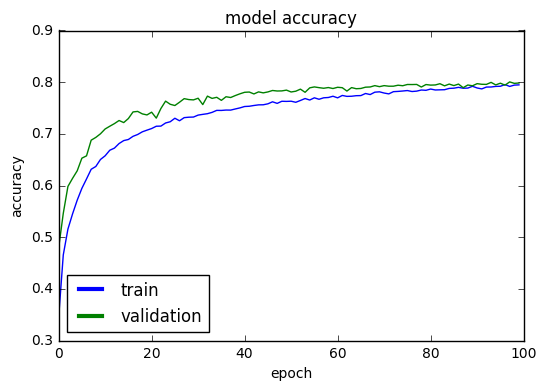# Object Recognition in CIFAR-10 Image Database¶CIFAR-10 is by now a classical computer-vision dataset for object recognition case study. It is a subset of the 80 million tiny images dataset that was designed and created by the Canadian Institute for Advanced Research (CIFAR, pronounced "see far").

The CIFAR-10 dataset consists of 60000 32x32x3 color images in 10 equal classes, (6000 images per class). Each class of images corresponds to a physical object (automobile, cat, dog, airplane, etc). It was collected by Alex Krizhevsky, Vinod Nair, and Geoffrey Hinton. We strongly recommend looking at the following two sources before starting work on this notebook:

## Prerequisites¶

The code of this IPython notebook run on Windows 10, Python 2.7 with keras, numpy, matplotlib and jupyter. We also use an NVIDIA GPU (GeForce GTX950) with cuDNN 5103. Of course, it can also be run on a CPU but it will be significantly slower (not recommended!).

To run the code in this notebook, you'll also need to download the following course libraries which we use in several examples of this course:

https://github.com/samyzaf/kerutils

In :
from keras.datasets import cifar10
from keras.preprocessing.image import ImageDataGenerator
from keras.layers import Dense, Dropout, Activation, Flatten
from keras.layers import Convolution2D, MaxPooling2D
from keras.optimizers import SGD
from keras.constraints import maxnorm
from keras.utils import np_utils
from keras.layers.noise import GaussianNoise
from keras.utils.visualize_util import plot
import pandas as pd
import matplotlib.pyplot as plt
import time, pickle
from kerutils import *
%matplotlib inline

Using Theano backend.
DEBUG: nvcc STDOUT mod.cu
Creating library C:/Users/samy/AppData/Local/Theano/compiledir_Windows-10-10.0.14393-Intel64_Family_6_Model_94_Stepping_3_GenuineIntel-2.7.11-64/tmpuhhmdp/265abc51f7c376c224983485238ff1a5.lib and object C:/Users/samy/AppData/Local/Theano/compiledir_Windows-10-10.0.14393-Intel64_Family_6_Model_94_Stepping_3_GenuineIntel-2.7.11-64/tmpuhhmdp/265abc51f7c376c224983485238ff1a5.exp

Using gpu device 0: GeForce GTX 950 (CNMeM is enabled with initial size: 80.0% of memory, cuDNN 5103)
c:\anaconda2\lib\site-packages\theano\sandbox\cuda\__init__.py:600: UserWarning: Your cuDNN version is more recent than the one Theano officially supports. If you see any problems, try updating Theano or downgrading cuDNN to version 5.
warnings.warn(warn)

In :
# These are css/html styles for good looking ipython notebooks
from IPython.core.display import HTML
HTML('<style>{}</style>'.format(css))

Out:

The CIFAR-10 image classes are encoded as integers 0-9 by the following Python dictionary

In :
nb_classes = 10
class_name = {
0: 'airplane',
1: 'automobile',
2: 'bird',
3: 'cat',
4: 'deer',
5: 'dog',
6: 'frog',
7: 'horse',
8: 'ship',
9: 'truck',
}


## Load training and test data¶

In :
(X_train, y_train), (X_test, y_test) = cifar10.load_data()
y_train = y_train.reshape(y_train.shape)  # somehow y_train comes as a 2D nx1 matrix
y_test = y_test.reshape(y_test.shape)

print('X_train shape:', X_train.shape)
print(X_train.shape, 'training samples)'
print(X_test.shape, 'validation samples)'

X_train shape: (50000L, 32L, 32L, 3L)
50000 training samples
10000 validation samples


The original data of each image is a 32x32x3 matrix of integers from 0 to 255. We need to scale it down to floats in the unit interval

In :
X_train = X_train.astype('float32')
X_test = X_test.astype('float32')
X_train /= 255
X_test /= 255


As usual, we must convert the y_train and y_test vectors to one-hot format:

0 → [1, 0, 0, 0, 0, 0, 0, 0, 0, 0]
1 → [0, 1, 0, 0, 0, 0, 0, 0, 0, 0]
2 → [0, 0, 1, 0, 0, 0, 0, 0, 0, 0]
3 → [0, 0, 0, 1, 0, 0, 0, 0, 0, 0]
etc...

In :
Y_train = np_utils.to_categorical(y_train, nb_classes)
Y_test = np_utils.to_categorical(y_test, nb_classes)


Let's also write two small utilities for drawing samples of images, so we can inspect our results visually.

In :
def draw_img(i):
im = X_train[i]
c = y_train[i]
plt.imshow(im)
plt.title("Class %d (%s)" % (c, class_name[c]))
plt.axis('on')

def draw_sample(X, y, n, rows=4, cols=4, imfile=None, fontsize=12):
for i in range(0, rows*cols):
plt.subplot(rows, cols, i+1)
im = X[n+i].reshape(32,32,3)
plt.imshow(im, cmap='gnuplot2')
plt.title("{}".format(class_name[y[n+i]]), fontsize=fontsize)
plt.axis('off')
if imfile:
plt.savefig(imfile)


Let's draw image 7 in X_train for example

In :
draw_img(7)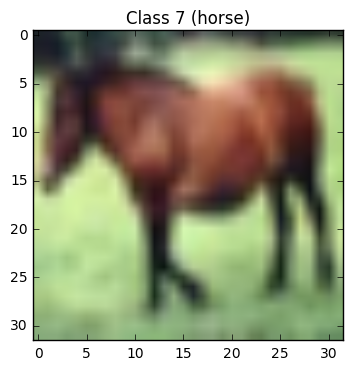To test the second utility, let's draw the first 15 images in a 3x5 grid:

In :
draw_sample(X_train, y_train, 0, 3, 5)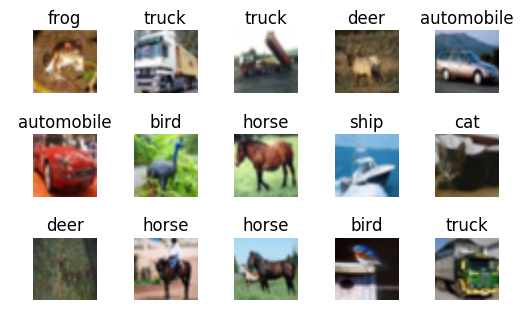In :
Y_train = np_utils.to_categorical(y_train, nb_classes)
Y_test = np_utils.to_categorical(y_test, nb_classes)


# Building Neural Networks for CIFAR-10¶

In contrast to previous case studies, in this case it would be prohibitive to use fully connected neural network unless we have good reasons to believe that we can make good progress with a small number of neurons on layers beyond the input layer. The input layer would have to be of size 3072 (as every image is a 32x32x3 matrix). if we add a hidden layer with the same size, we'll end up with 9 milion synapses on the first floor. Adding one more layer of such size will take us to billions of synapses, which is of course impractical.

Deep learning frameworks have come up with special types of designated layers for processing images with minimal number of synapses (compared to Dense layer). Each image pixel is connected to a very small subset of pixels of size 3x3 or 5x5 in its neighborhood. Intuitively, image pixels are mostly impacted by pixels around them rather than pixels in a far away region of the image.

These two types of layers are explained in more detail in the following two articles, which we recommend to read before you approach the following code:

We will start with a small Keras model which combines a well thought mix of Convolution2D, Maxpooling2D and Dense layers. It is mostly based on open source code examples by François Chollet (author of Keras from Google) and other similar sources:

## Two Types of Training¶

We will use two types of training:

1. Standard training: the usual Keras fit method
2. Training with augmented data: In this mode, our training data is passing through a special Keras generator which applies certain image operations on each data item and generates new items for training. This way we can multiply our training data indefinitely as much as we wish and thus provide our model with as much training as we wish (but of course we should avoid overfitting).

The Keras generator for the second training mode is called ImageDataGenerator and can be understood from the Keras manual page:
https://keras.io/preprocessing/image/#imagedatagenerator

## Lets Train Model 1 (standard training)¶

In :
nb_epoch = 50
batch_size = 32

model1 = Sequential()
model1.add(Convolution2D(32, 3, 3, input_shape=(32, 32, 3), border_mode='same', activation='relu', W_constraint=maxnorm(3)))
model1.add(Convolution2D(32, 3, 3, activation='relu', border_mode='same', W_constraint=maxnorm(3)))
# Compile model
lrate = 0.01
decay = lrate/nb_epoch
sgd = SGD(lr=lrate, momentum=0.9, decay=decay, nesterov=False)
model1.compile(loss='categorical_crossentropy', optimizer=sgd, metrics=['accuracy'])
print(model1.summary())

print('Standard Training.')

h = model1.fit(
X_train,
Y_train,
batch_size=batch_size,
nb_epoch=nb_epoch,
validation_data=(X_test, Y_test),
shuffle=True
)

show_scores(model1, h, X_train, Y_train, X_test, Y_test)
print('Saving model1 to the file "model1.h5"')
model1.save("model1.h5")

Model Summary:
____________________________________________________________________________________________________
Layer (type)                     Output Shape          Param #     Connected to
====================================================================================================
convolution2d_3 (Convolution2D)  (None, 32, 32, 32)    896         convolution2d_input_2
____________________________________________________________________________________________________
dropout_3 (Dropout)              (None, 32, 32, 32)    0           convolution2d_3
____________________________________________________________________________________________________
convolution2d_4 (Convolution2D)  (None, 32, 32, 32)    9248        dropout_3
____________________________________________________________________________________________________
maxpooling2d_2 (MaxPooling2D)    (None, 16, 16, 32)    0           convolution2d_4
____________________________________________________________________________________________________
flatten_2 (Flatten)              (None, 8192)          0           maxpooling2d_2
____________________________________________________________________________________________________
dense_3 (Dense)                  (None, 512)           4194816     flatten_2
____________________________________________________________________________________________________
dropout_4 (Dropout)              (None, 512)           0           dense_3
____________________________________________________________________________________________________
dense_4 (Dense)                  (None, 10)            5130        dropout_4
====================================================================================================
Total params: 4210090
____________________________________________________________________________________________________
None
Standard Training.
Train on 50000 samples, validate on 10000 samples
Epoch 1/50
50000/50000 [==============================] - 34s - loss: 1.7511 - acc: 0.3645 - val_loss: 1.4763 - val_acc: 0.4601
Epoch 2/50
50000/50000 [==============================] - 34s - loss: 1.4102 - acc: 0.4927 - val_loss: 1.2470 - val_acc: 0.5588
Epoch 3/50
50000/50000 [==============================] - 34s - loss: 1.2466 - acc: 0.5534 - val_loss: 1.1544 - val_acc: 0.5939
Epoch 4/50
50000/50000 [==============================] - 34s - loss: 1.1335 - acc: 0.5971 - val_loss: 1.1024 - val_acc: 0.6102
Epoch 5/50
50000/50000 [==============================] - 34s - loss: 1.0310 - acc: 0.6337 - val_loss: 1.0466 - val_acc: 0.6321
Epoch 6/50
50000/50000 [==============================] - 34s - loss: 0.9422 - acc: 0.6659 - val_loss: 1.0070 - val_acc: 0.6489
Epoch 7/50
50000/50000 [==============================] - 34s - loss: 0.8644 - acc: 0.6940 - val_loss: 1.0108 - val_acc: 0.6482
Epoch 8/50
50000/50000 [==============================] - 35s - loss: 0.7860 - acc: 0.7201 - val_loss: 0.9407 - val_acc: 0.6727
Epoch 9/50
50000/50000 [==============================] - 34s - loss: 0.7211 - acc: 0.7420 - val_loss: 0.9388 - val_acc: 0.6803
Epoch 10/50
50000/50000 [==============================] - 34s - loss: 0.6512 - acc: 0.7714 - val_loss: 0.9561 - val_acc: 0.6750
Epoch 11/50
50000/50000 [==============================] - 34s - loss: 0.6022 - acc: 0.7869 - val_loss: 0.9649 - val_acc: 0.6781
Epoch 12/50
50000/50000 [==============================] - 34s - loss: 0.5512 - acc: 0.8027 - val_loss: 0.9646 - val_acc: 0.6828
Epoch 13/50
50000/50000 [==============================] - 34s - loss: 0.5062 - acc: 0.8198 - val_loss: 0.9558 - val_acc: 0.6915
Epoch 14/50
50000/50000 [==============================] - 34s - loss: 0.4622 - acc: 0.8348 - val_loss: 0.9656 - val_acc: 0.6870
Epoch 15/50
50000/50000 [==============================] - 34s - loss: 0.4208 - acc: 0.8499 - val_loss: 0.9934 - val_acc: 0.6902
Epoch 16/50
50000/50000 [==============================] - 34s - loss: 0.3878 - acc: 0.8624 - val_loss: 1.0046 - val_acc: 0.6924
Epoch 17/50
50000/50000 [==============================] - 34s - loss: 0.3639 - acc: 0.8704 - val_loss: 1.0410 - val_acc: 0.6898
Epoch 18/50
50000/50000 [==============================] - 34s - loss: 0.3318 - acc: 0.8824 - val_loss: 1.0516 - val_acc: 0.6917
Epoch 19/50
50000/50000 [==============================] - 34s - loss: 0.3084 - acc: 0.8897 - val_loss: 1.0763 - val_acc: 0.6924
Epoch 20/50
50000/50000 [==============================] - 34s - loss: 0.2865 - acc: 0.8982 - val_loss: 1.1012 - val_acc: 0.6929
Epoch 21/50
50000/50000 [==============================] - 34s - loss: 0.2690 - acc: 0.9031 - val_loss: 1.0899 - val_acc: 0.6914
Epoch 22/50
50000/50000 [==============================] - 34s - loss: 0.2495 - acc: 0.9108 - val_loss: 1.1001 - val_acc: 0.6964
Epoch 23/50
50000/50000 [==============================] - 34s - loss: 0.2342 - acc: 0.9170 - val_loss: 1.1171 - val_acc: 0.7017
Epoch 24/50
50000/50000 [==============================] - 34s - loss: 0.2208 - acc: 0.9220 - val_loss: 1.1430 - val_acc: 0.6965
Epoch 25/50
50000/50000 [==============================] - 34s - loss: 0.2097 - acc: 0.9262 - val_loss: 1.1811 - val_acc: 0.6965
Epoch 26/50
50000/50000 [==============================] - 34s - loss: 0.1950 - acc: 0.9320 - val_loss: 1.1600 - val_acc: 0.7006
Epoch 27/50
50000/50000 [==============================] - 34s - loss: 0.1886 - acc: 0.9337 - val_loss: 1.2166 - val_acc: 0.6974
Epoch 28/50
50000/50000 [==============================] - 34s - loss: 0.1749 - acc: 0.9380 - val_loss: 1.2317 - val_acc: 0.7014
Epoch 29/50
50000/50000 [==============================] - 34s - loss: 0.1692 - acc: 0.9409 - val_loss: 1.2404 - val_acc: 0.6948
Epoch 30/50
50000/50000 [==============================] - 34s - loss: 0.1652 - acc: 0.9427 - val_loss: 1.2466 - val_acc: 0.6998
Epoch 31/50
50000/50000 [==============================] - 34s - loss: 0.1571 - acc: 0.9458 - val_loss: 1.2328 - val_acc: 0.6991
Epoch 32/50
50000/50000 [==============================] - 34s - loss: 0.1461 - acc: 0.9486 - val_loss: 1.2797 - val_acc: 0.6958
Epoch 33/50
50000/50000 [==============================] - 34s - loss: 0.1428 - acc: 0.9498 - val_loss: 1.2880 - val_acc: 0.6939
Epoch 34/50
50000/50000 [==============================] - 34s - loss: 0.1356 - acc: 0.9539 - val_loss: 1.2841 - val_acc: 0.6989
Epoch 35/50
50000/50000 [==============================] - 34s - loss: 0.1327 - acc: 0.9544 - val_loss: 1.2901 - val_acc: 0.6989
Epoch 36/50
50000/50000 [==============================] - 34s - loss: 0.1265 - acc: 0.9569 - val_loss: 1.3254 - val_acc: 0.6971
Epoch 37/50
50000/50000 [==============================] - 34s - loss: 0.1212 - acc: 0.9590 - val_loss: 1.3383 - val_acc: 0.6995
Epoch 38/50
50000/50000 [==============================] - 34s - loss: 0.1177 - acc: 0.9589 - val_loss: 1.3340 - val_acc: 0.7019
Epoch 39/50
50000/50000 [==============================] - 34s - loss: 0.1151 - acc: 0.9600 - val_loss: 1.3548 - val_acc: 0.7027
Epoch 40/50
50000/50000 [==============================] - 34s - loss: 0.1104 - acc: 0.9621 - val_loss: 1.3613 - val_acc: 0.7033
Epoch 41/50
50000/50000 [==============================] - 34s - loss: 0.1088 - acc: 0.9629 - val_loss: 1.3798 - val_acc: 0.6991
Epoch 42/50
50000/50000 [==============================] - 34s - loss: 0.1040 - acc: 0.9653 - val_loss: 1.3835 - val_acc: 0.7005
Epoch 43/50
50000/50000 [==============================] - 34s - loss: 0.1016 - acc: 0.9658 - val_loss: 1.3968 - val_acc: 0.7028
Epoch 44/50
50000/50000 [==============================] - 34s - loss: 0.0954 - acc: 0.9682 - val_loss: 1.3809 - val_acc: 0.7025
Epoch 45/50
50000/50000 [==============================] - 34s - loss: 0.0987 - acc: 0.9662 - val_loss: 1.3955 - val_acc: 0.7025
Epoch 46/50
50000/50000 [==============================] - 34s - loss: 0.0958 - acc: 0.9682 - val_loss: 1.4032 - val_acc: 0.7032
Epoch 47/50
50000/50000 [==============================] - 34s - loss: 0.0896 - acc: 0.9699 - val_loss: 1.4049 - val_acc: 0.7035
Epoch 48/50
50000/50000 [==============================] - 34s - loss: 0.0857 - acc: 0.9713 - val_loss: 1.3944 - val_acc: 0.7009
Epoch 49/50
50000/50000 [==============================] - 34s - loss: 0.0889 - acc: 0.9703 - val_loss: 1.4177 - val_acc: 0.7050
Epoch 50/50
50000/50000 [==============================] - 34s - loss: 0.0870 - acc: 0.9704 - val_loss: 1.4261 - val_acc: 0.7014
Training: accuracy   = 0.999560 loss = 0.005692
Validation: accuracy = 0.701400 loss = 1.426094
Over fitting score   = 0.236352
Under fitting score  = 0.191977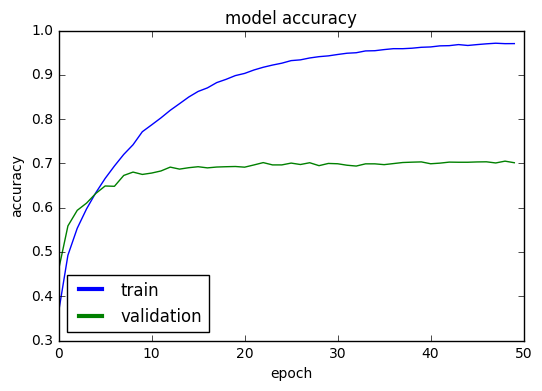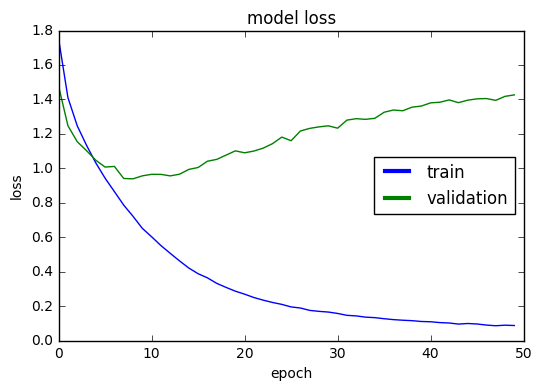In :
loss, accuracy = model1.evaluate(X_train, Y_train, verbose=0)
print("Training: accuracy = %f  ;  loss = %f" % (accuracy, loss))

Training: accuracy = 0.999560  ;  loss = 0.005692

In :
loss, accuracy = model1.evaluate(X_test, Y_test, verbose=0)
print("Validation: accuracy1 = %f  ;  loss1 = %f" % (accuracy, loss))

Validation: accuracy1 = 0.701400  ;  loss1 = 1.426094


What we see in the last two graphs is a classic example of overfitting phenomenon. While the training accuracy has skyrocketed to 99.96% (wow!!), our validation data comes to the rescue and cools down our enthusiasm: only 70.14%. The almost 30% gap between the training data and validation data is a clear indication of overfitting, and a good reason to abandone model1 and look for a better one. We should also notice the clear big gap between the training loss and validation loss. This is also a clear mark f overfitting that should raise a warning sign.

## Inspecting the output¶

Neverthelss, befor we search for a new model, let's take a quick look on some of the cases that our model1 missed. It may give us hints on the strengths an weaknesses of NN models, and what we can expect from these artificial models.

The predict_classes method is helpful for getting a vector (y_pred) of the predicted classes of model1. We should compare y_pred to the expected true classes y_test in order to get the false cases:

In :
y_pred = model1.predict_classes(X_test)

 9984/10000 [============================>.] - ETA: 0s
In :
true_preds = [(x,y) for (x,y,p) in zip(X_test, y_test, y_pred) if y == p]
false_preds = [(x,y,p) for (x,y,p) in zip(X_test, y_test, y_pred) if y != p]
print("Number of true predictions: ", len(true_preds))
print("Number of false predictions:", len(false_preds))

Number of true predictions:  7014
Number of false predictions: 2986


The array false_preds consists of all triples (x,y,p) where x is an image, y is its true class, and p is the false predicted value of model1.

Lets visualize a sample of 15 items:

In :
for i,(x,y,p) in enumerate(false_preds[0:15]):
plt.subplot(3, 5, i+1)
plt.imshow(x, cmap='gnuplot2')
plt.title("y: %s\np: %s" % (class_name[y], class_name[p]), fontsize=9, loc='left')
plt.axis('off')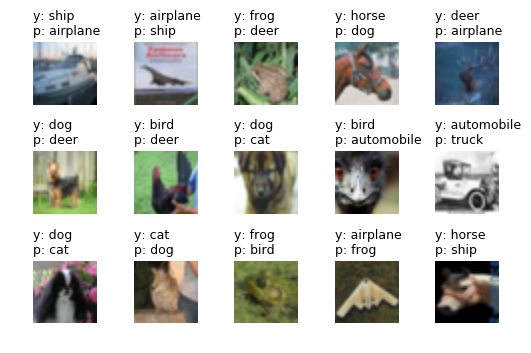Well, we see that model1 confuses between airplanes and sheep, dogs and cats, etc. But we should not underestimate the fact that it is still correct in 70% of the cases, which is highly untrivial! (suppose that as a programmer you were assigned to write a traditional computer program that can guess the class in 70% of the case - think how hard it would be...)

## Second Keras Model for the CIFAR-10 dataset¶

Lets try our small model with the aid of augmented data. The Keras ImageDataGenerator is a great tool for generating more training data from old data, so that we may have enough training and avoid overfitting.

The ImageDataGenerator takes quite a few graphic parameters which we cannot explain in this tutorial. We recommend reading the Keras documentation page and a short tutorial:

Lets first take a look at a few samples of images that are genereated but ImageDataGenerator:

In :
imdgen = ImageDataGenerator(
featurewise_center = False,  # set input mean to 0 over the dataset
samplewise_center = False,  # set each sample mean to 0
featurewise_std_normalization = False,  # divide inputs by std of the dataset
samplewise_std_normalization = False,  # divide each input by its std
zca_whitening = False,  # apply ZCA whitening
rotation_range = 0,  # randomly rotate images in the range (degrees, 0 to 180)
width_shift_range = 0.1,  # randomly shift images horizontally (fraction of total width)
height_shift_range = 0.1,  # randomly shift images vertically (fraction of total height)
horizontal_flip = True,  # randomly flip images
vertical_flip = False,  # randomly flip images
)

imdgen.fit(X_train)
it = imdgen.flow(X_train, Y_train, batch_size=15) # This is a Python iterator
images, categories = it.next()
print("Number of images returned by iterator:", len(images))
for i in range(15):
plt.subplot(3, 5, i+1)
im = images[i]
c = np.where(categories[i] == 1) # convert one-hot to regular index
plt.imshow(im, cmap='gnuplot2')
plt.title(class_name[c], fontsize=9)
plt.axis('off')

Number of images returned by iterator: 15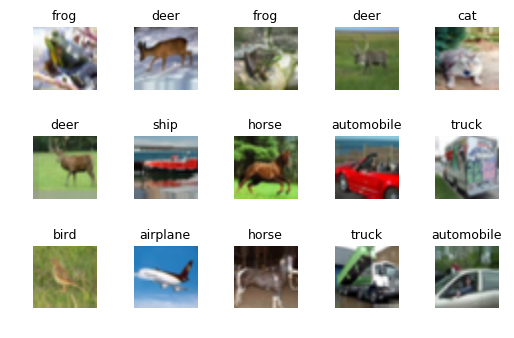The images you see are not from the CIFAR-10 collection. They were generated by Keras ImageDataGenerator from images in the CIFAR-10 database by applying various image operators on them. This way we can increase the number of training samples almost indefinitely (in every training epoch we get a completely new set of samples!)

The second important point to note about this iterator is that it does not require any memory or disk space to keep its images (no matter how many of them we want to make)! It generates them in small batches (usually 32 or 128 at a time), and they are discarded after model training. So we can train our model with millions of samples without using a memory more than 100KB (for 32 batch size) or 400KB (for 128 batch size). This is extremely important when our images are in real size (like 2048x3072).

Lets see now the second type of Keras training based on the ImageDataGenerator. Note the new training method name: fit_generator.

### Model 2 (with Data Augmentation)¶

In :
nb_epoch = 100   # This tim lets increase the number of epochs to 100
batch_size = 32

model2 = Sequential()
model2.add(Convolution2D(32, 3, 3, input_shape=(32, 32, 3), border_mode='same', activation='relu', W_constraint=maxnorm(3)))
model2.add(Convolution2D(32, 3, 3, activation='relu', border_mode='same', W_constraint=maxnorm(3)))
# Compile model with SGD
lrate = 0.01
decay = lrate/nb_epoch
sgd = SGD(lr=lrate, momentum=0.9, decay=decay, nesterov=False)
model2.compile(loss='categorical_crossentropy', optimizer=sgd, metrics=['accuracy'])
print(model2.summary())

print('Augmented Data Training.')

imdgen = ImageDataGenerator(
featurewise_center = False,  # set input mean to 0 over the dataset
samplewise_center = False,  # set each sample mean to 0
featurewise_std_normalization = False,  # divide inputs by std of the dataset
samplewise_std_normalization = False,  # divide each input by its std
zca_whitening = False,  # apply ZCA whitening
rotation_range = 0,  # randomly rotate images in the range (degrees, 0 to 180)
width_shift_range = 0.1,  # randomly shift images horizontally (fraction of total width)
height_shift_range = 0.1,  # randomly shift images vertically (fraction of total height)
horizontal_flip = True,  # randomly flip images
vertical_flip = False,  # randomly flip images
)

# compute quantities required for featurewise normalization
# (std, mean, and principal components if ZCA whitening is applied)
imdgen.fit(X_train)

# fit the model on the batches generated by datagen.flow()
dgen = imdgen.flow(X_train, Y_train, batch_size=batch_size)
fmon = FitMonitor(thresh=0.03, minacc=0.98)  # this is from our kerutils module (see above)
h = model2.fit_generator(
dgen,
samples_per_epoch = X_train.shape,
nb_epoch = nb_epoch,
validation_data = (X_test, Y_test),
verbose = 0,
callbacks = [fmon]
)

show_scores(model2, h, X_train, Y_train, X_test, Y_test)
print('Saving model2 to "model2.h5"')
model2.save("model2.h5")

____________________________________________________________________________________________________
Layer (type)                     Output Shape          Param #     Connected to
====================================================================================================
convolution2d_3 (Convolution2D)  (None, 32, 32, 32)    896         convolution2d_input_2
____________________________________________________________________________________________________
dropout_3 (Dropout)              (None, 32, 32, 32)    0           convolution2d_3
____________________________________________________________________________________________________
convolution2d_4 (Convolution2D)  (None, 32, 32, 32)    9248        dropout_3
____________________________________________________________________________________________________
maxpooling2d_2 (MaxPooling2D)    (None, 16, 16, 32)    0           convolution2d_4
____________________________________________________________________________________________________
flatten_2 (Flatten)              (None, 8192)          0           maxpooling2d_2
____________________________________________________________________________________________________
dense_3 (Dense)                  (None, 512)           4194816     flatten_2
____________________________________________________________________________________________________
dropout_4 (Dropout)              (None, 512)           0           dense_3
____________________________________________________________________________________________________
dense_4 (Dense)                  (None, 10)            5130        dropout_4
====================================================================================================
Total params: 4210090
____________________________________________________________________________________________________
None
Augmented Data Training.
Train begin: 2016-11-26 16:06:54
Stop file: stop_training_file.keras (create this file to stop training gracefully)
Pause file: pause_training_file.keras (create this file to pause training and view graphs)
do_validation = True
metrics = ['loss', 'acc', 'val_loss', 'val_acc']
nb_epoch = 100
nb_sample = 50000
verbose = 0
..... 05% epoch=5 acc=0.594480 loss=1.139003 val_acc=0.652900 val_loss=0.968054
max_acc=0.594480 max_val_acc=0.652900
..... 10% epoch=10 acc=0.657480 loss=0.969157 val_acc=0.709400 val_loss=0.825718
max_acc=0.657480 max_val_acc=0.709400
..... 15% epoch=15 acc=0.689060 loss=0.878997 val_acc=0.729600 val_loss=0.773610
max_acc=0.689060 max_val_acc=0.729600
..... 20% epoch=20 acc=0.710240 loss=0.826102 val_acc=0.741900 val_loss=0.727546
max_acc=0.710240 max_val_acc=0.743400
..... 25% epoch=25 acc=0.730160 loss=0.777298 val_acc=0.754800 val_loss=0.701633
max_acc=0.730160 max_val_acc=0.763300
..... 30% epoch=30 acc=0.736260 loss=0.749473 val_acc=0.768900 val_loss=0.665305
max_acc=0.736260 max_val_acc=0.768900
..... 35% epoch=35 acc=0.745280 loss=0.729686 val_acc=0.764800 val_loss=0.667546
max_acc=0.745400 max_val_acc=0.773000
..... 40% epoch=40 acc=0.752900 loss=0.706349 val_acc=0.780400 val_loss=0.645095
max_acc=0.752900 max_val_acc=0.780400
..... 45% epoch=45 acc=0.758080 loss=0.689673 val_acc=0.781100 val_loss=0.638592
max_acc=0.758080 max_val_acc=0.781200
..... 50% epoch=50 acc=0.763140 loss=0.671631 val_acc=0.781000 val_loss=0.634494
max_acc=0.763140 max_val_acc=0.784600
..... 55% epoch=55 acc=0.769720 loss=0.662885 val_acc=0.790800 val_loss=0.614884
max_acc=0.769720 max_val_acc=0.790800
..... 60% epoch=60 acc=0.769640 loss=0.652074 val_acc=0.790100 val_loss=0.609898
max_acc=0.772760 max_val_acc=0.790800
..... 65% epoch=65 acc=0.774020 loss=0.640879 val_acc=0.787800 val_loss=0.617783
max_acc=0.774280 max_val_acc=0.790800
..... 70% epoch=70 acc=0.778960 loss=0.624706 val_acc=0.793300 val_loss=0.609352
max_acc=0.781320 max_val_acc=0.793300
..... 75% epoch=75 acc=0.783760 loss=0.614971 val_acc=0.795500 val_loss=0.608315
max_acc=0.783760 max_val_acc=0.795500
..... 80% epoch=80 acc=0.786700 loss=0.609307 val_acc=0.794300 val_loss=0.599971
max_acc=0.786700 max_val_acc=0.795600
..... 85% epoch=85 acc=0.788340 loss=0.602530 val_acc=0.793400 val_loss=0.603756
max_acc=0.788340 max_val_acc=0.797000
..... 90% epoch=90 acc=0.788760 loss=0.603021 val_acc=0.797100 val_loss=0.599524
max_acc=0.792060 max_val_acc=0.797100
..... 95% epoch=95 acc=0.792060 loss=0.593095 val_acc=0.798000 val_loss=0.597773
max_acc=0.792060 max_val_acc=0.799600
.... 99% epoch=99 acc=0.794760 loss=0.584867
Train end: 2016-11-26 17:01:36
Total run time: 3282.28 seconds
max_acc = 0.795400  epoc = 96
max_val_acc = 0.800500  epoc = 97
Training: accuracy   = 0.884280 loss = 0.346051
Validation: accuracy = 0.798400 loss = 0.593385
Over fitting score   = 0.015105
Under fitting score  = 0.025015